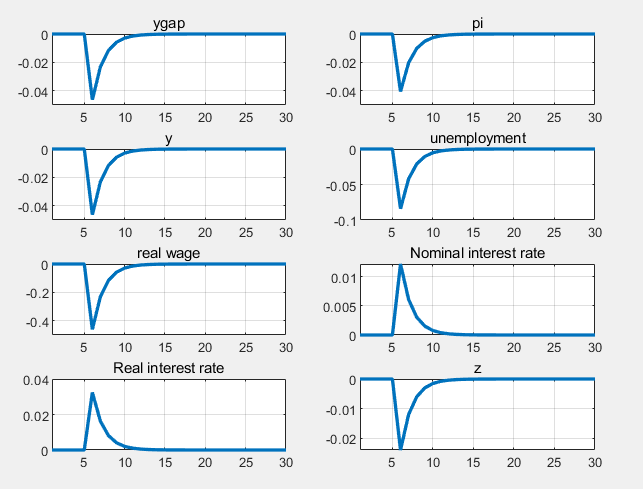# ZLB with OCCBIN

hi, when I use the occbin toolbox to solve ZLB, my model is a simple NK model, the model is set to linear, so that my steady state value is 0, and the impact of the variables in the previous periods are 0. I think something is wrong, but I dont know where can I modify? What should I do? I see that the code provided by the author is non-linear, so adding a steady state value can draw a normal graph.
Many thanks.like this image, the shock of all variables starts at 0. I referenced the occbin source code，the graph code is “plot(BETA*(ut_p+ut_ss),‘k’)”, author added steady state value, So his graph like. But my model is linear, so the steady state value is zero.